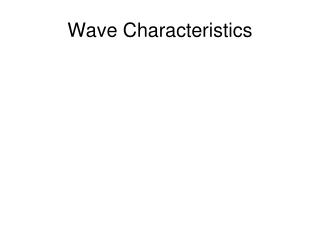DownloadDownload PresentationWave Characteristics

# Wave Characteristics

Télécharger la présentation## Wave Characteristics

- - - - - - - - - - - - - - - - - - - - - - - - - - - E N D - - - - - - - - - - - - - - - - - - - - - - - - - - -
##### Presentation Transcript

1. Wave Characteristics

2. Waves

3. Waves A disturbance that carries energy through matter or space.

4. Medium The matter through which a wave travels

5. Mechanical Waves Waves that require a medium in which to travel.

6. Longitudinal Waves Waves in which the motion of the particles is parallel to the motion of the wave as a whole.

7. Longitudinal Waves Also called a compressional wave

8. Electromagnetic Waves A wave that travels at the speed of light. It travels by oscillating electric and magnetic fields. 3.0 X 108 m/s

9. Transverse Waves Waves in which the motion of the particles is perpendicular to the motion of the wave as a whole

10. Properties of Waves • Crest • Trough • Amplitude • Wavelength • Period • Frequency

11. Period The time that it takes a complete cycle or wave oscillation to occur (T)

12. Frequency The number of cycles or vibration per unit of time f=1/T (measure in Hertz (Hz))

13. Electromagnetic Spectrum All of the frequencies or wavelengths of electromagnetic radiation.

14. Wave speed is found to be the ratio of wavelength to the period. Wave speed is found to be the frequency times wavelength.

15. Reflection and Refraction

16. Reflection The bouncing back of a wave when the wave hits a surface

17. Normal An imaginary line that is perpendicular to the surface.

18. Angle of Incidence The angle of the light rays hitting the surface. It is measured from the normal.

19. Angle of Reflection The angle of the light rays reflecting off the surface. It is measured from the normal.

20. Diffraction A change in the direction of a wave when the wave finds an obstacle or an edge, such as on opening

21. Refraction The bending of a wavefront as the wavefront passes between two substances in which the speed of the wafe differs.

22. Dispersion In optics, the process of separating a wave of different frequencies into its individual component waves.

23. Interference The combination of two or more waves that results in a single wave

24. Two type of Interference • Constructive Interference • Destructive Interference

25. Standing Wave A pattern of vibration that simulates a wave that is standing still

26. Nodes A point in a standing wave that maintains zero amplitude

27. Antinode A point in a standing wave, halfway between two nodes, it indicates a position of maximum intensity.

28. Sound

29. Sound waves are longitudinal wavesSpeed of sound346 m/s

30. Pitch Measure of how high or low a sound is depending on the frequency.

31. Beat The repeating changes in loudness of two sound waves that are interfering Beat=f1-f2

32. Doppler Effect An observed change in frequency of a wave when the source or observer is moving. When objects get closer the frequency increases and has the distance increases then the frequency decreases.

33. Infrasound Slow vibrations of frequencies lower than 20 Hz

34. Ultrasound Any sound wave with frequencies higher than 20000 Hz

35. Intensity of sound is measure in decibels (dB). Humans can hear from 0dB to 120dB. 120 dB is the point when the human hear has pain. Damage starts at 85 dB. 10dB equals twice the loudness.

36. Resonance A phenomenon that occurs when two objects naturally vibrate at the same frequency.

37. Natural Frequency The frequency which causes a substance to vibrate.

38. SONAR Sound Navigation and Ranging; a system that uses acoustic signals and echo returns to determine the location of objects or to communicate.